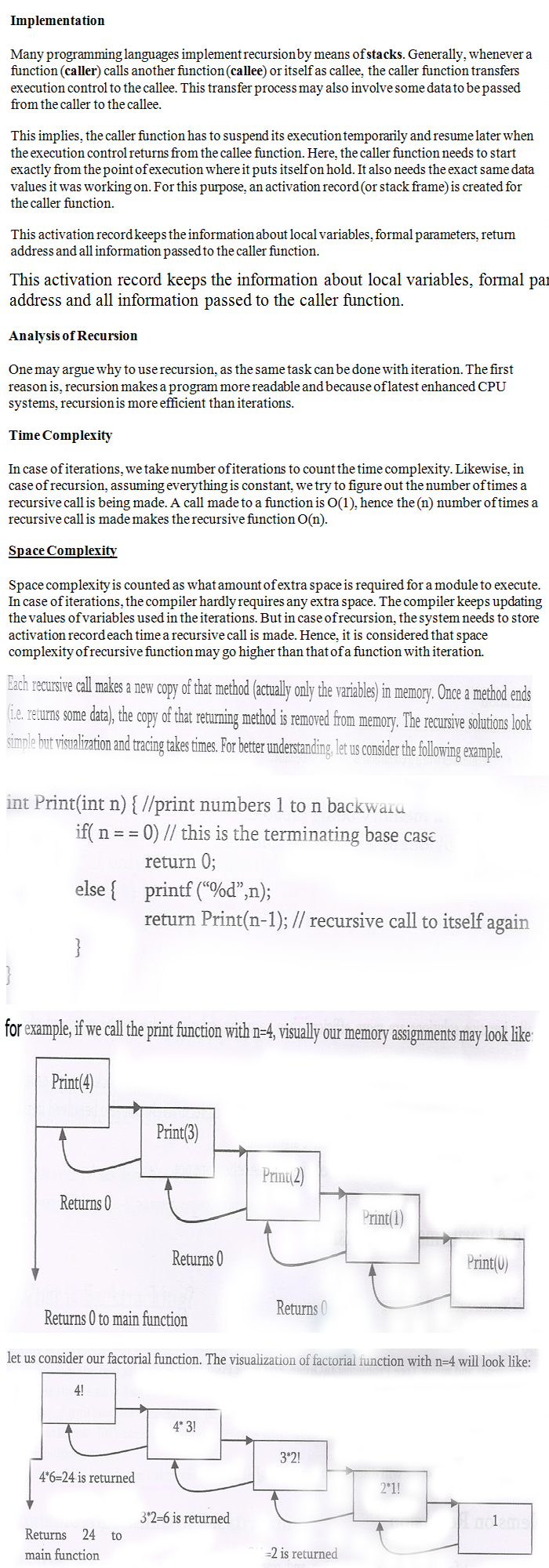## DESIGN AND ANALYSIS OF ALGORITHM

Basic definition of Recursion and Examples
14 November, 2016

Recursion:  Any function which calls itself is called recursive. A recursive method solves a problem by calling a copy of itself to work on a smaller problem.

In recursion, a function α either calls itself directly or calls a function β that in turn calls the original function α. The function α is called recursive function. This is called recursion step. The recursion step can result in many more such recursive calls. It is important to ensure that the recursion terminates.

Example1 − a function calling itself.

int function(int value) {

if(value < 1)
return;

function(value - 1);

printf("%d ",value);
}

Example2 − a function that calls another function which in turn calls it again.

int function(int value) {

if(value < 1)
return;
function(value - 1);

printf("%d ",value);
}

Note: Both works almost same.

Importance of recursion: Recursion is a useful technique borrowed from maths. Recursive code is generally shorter and easier to write than iterative code. Generally , loops are turned into recursive functions when they are compiled or interpreted.

Notes on Recursion:

• Recursive algorithms have two types of cases, recursive cases and base cases.
• Every recursive function case must terminates at base case.
• Iterative solutions are more efficient than Recursive.
• A recursive algorithm can be implemented without recursive functions calls using a stack, but its usually more trouble than its worth. That means any problem that can be solved recursively can also be solved iteration.Examples based on recursion:

•      Tower of hanoi
•      Divide and conquer Algorithms
•      Dynamic Programming Examples
•      Graph Traversals
•     Tree Traversals
•     Binary search
•     Merge Sort, Quick Sort
•     Fibonacci Series, Factorial Finding
•     Backtracking Algorithms